# matplotlib.axes.Axes.set_xlim¶

Axes.set_xlim(left=None, right=None, emit=True, auto=False, *, xmin=None, xmax=None)[source]

Set the x-axis view limits.

Parameters
leftfloat, optional

The left xlim in data coordinates. Passing None leaves the limit unchanged.

The left and right xlims may also be passed as the tuple (left, right) as the first positional argument (or as the left keyword argument).

rightfloat, optional

The right xlim in data coordinates. Passing None leaves the limit unchanged.

emitbool, default: True

Whether to notify observers of limit change.

autobool or None, default: False

Whether to turn on autoscaling of the x-axis. True turns on, False turns off, None leaves unchanged.

xmin, xmaxfloat, optional

They are equivalent to left and right respectively, and it is an error to pass both xmin and left or xmax and right.

Returns
left, right(float, float)

The new x-axis limits in data coordinates.

Notes

The left value may be greater than the right value, in which case the x-axis values will decrease from left to right.

Examples

```>>> set_xlim(left, right)
>>> set_xlim((left, right))
>>> left, right = set_xlim(left, right)
```

One limit may be left unchanged.

```>>> set_xlim(right=right_lim)
```

Limits may be passed in reverse order to flip the direction of the x-axis. For example, suppose x represents the number of years before present. The x-axis limits might be set like the following so 5000 years ago is on the left of the plot and the present is on the right.

```>>> set_xlim(5000, 0)
```

## Examples using `matplotlib.axes.Axes.set_xlim`¶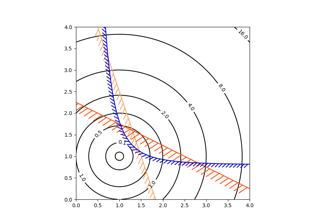Contouring the solution space of optimizations¶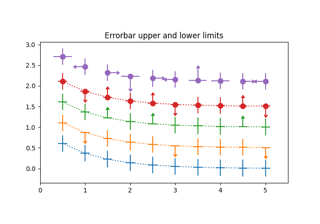Including upper and lower limits in error bars¶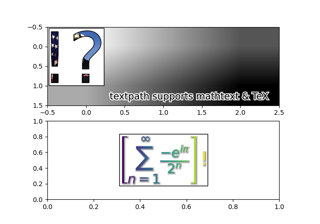Using a text as a Path¶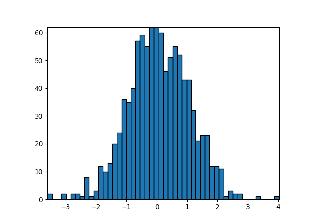Building histograms using Rectangles and PolyCollections¶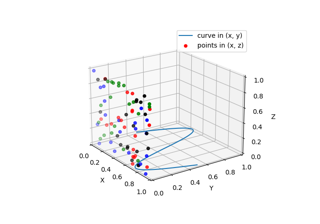Plot 2D data on 3D plot¶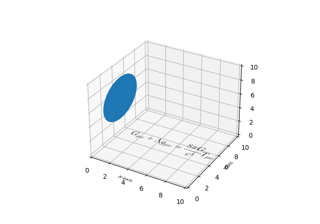Draw flat objects in 3D plot¶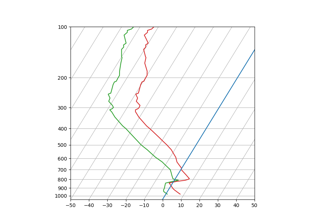SkewT-logP diagram: using transforms and custom projections¶